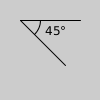# Py5Vector.angle_between()#

Measure the angle between two vectors.

## Examples#

import numpy as np

v1 = py5.Py5Vector(0, 40)
v2 = py5.Py5Vector(10, 10)
angle = v1.angle_between(v2)
print(f'angle = {round(py5.degrees(angle))}°')
# angle = 45°
angle = np.arccos(v1.norm.dot(v2.norm))
print(f'angle = {round(py5.degrees(angle))}°')
# angle = 45°def setup():
py5.translate(20, 20)
v1 = py5.Py5Vector(60, 0)
v2 = py5.Py5Vector(45, 45)
py5.line(0, 0, v1.x, v1.y)
py5.line(0, 0, v2.x, v2.y)
py5.no_fill()
py5.arc(0, 0, 40, 40, 0, angle_radians)
py5.fill(0)


## Description#

Measure the angle between two vectors.

## Signatures#

angle_between(
other: Union[Py5Vector, np.ndarray]  # vector to measure angle between
) -> Union[float, np.ndarray[np.floating]]


Updated on March 06, 2023 02:49:26am UTC